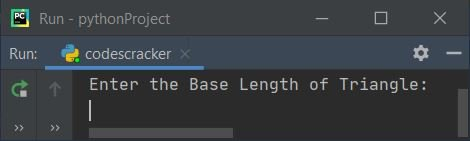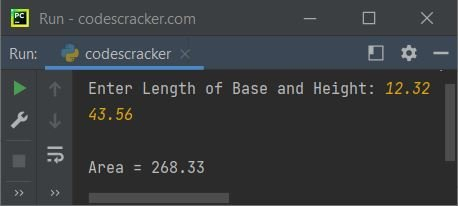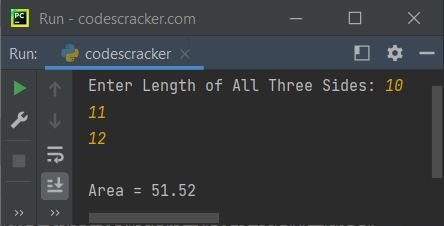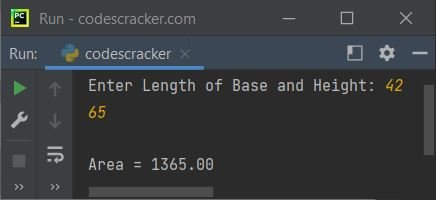# Python Program to Find Area of Triangle

In this article, we've listed some programs in Python, to find and print area of triangle. Here are the list of programs available in this article:

• Find Area of Triangle based on Base and Height
• Find Area of Triangle based on Three Sides
• Find Area of Triangle using user-defined Function
• using Class and Object

Note - Before starting these programs, if you're not aware about some formulae used to do the task of finding area of triangle, refer to Area of Triangle Formula to get every required things about the topic.

## Find Area of Triangle based on Base and Height

This program finds area of triangle based on base and height value entered by user at run-time. The question is, write a Python program to find area of triangle with base and height value. Here is its answer:

```print("Enter the Base Length of Triangle: ")
b = float(input())
print("Enter the Height Length Triangle: ")
h = float(input())
a = 0.5 * b * h
print("\nArea = ", a)```

Here is its initial output:Now supply inputs say 5 as length of base and 4 as length of height (perpendicular line from base) of triangle, then press `ENTER` key to find and print its area as shown in the snapshot given below:#### Modified Version of Previous Program

This is the modified version of previous program. In this program, we've used end= to skip an automatic printing of newline using print(). And {:.2f} is used to print a floating-point value upto only 2 decimal places using format() method attached with it as given in this program.

```print("Enter Length of Base and Height: ", end="")
b = float(input())
h = float(input())
print("\nArea = {:.2f}".format(0.5*b*h))```

Here is its sample run with user input 12.32 as base and 43.56 as height of triangle:## Find Area of Triangle based on Three Sides

To find area of a triangle (based on three sides) in Python, you have to ask from user to enter the length of first, second, and third sides of triangle. Now calculate and print the area value of that triangle as shown in the program given below.

```import math
print("Enter Length of All Three Sides: ", end="")
a = float(input())
b = float(input())
c = float(input())
s = (a+b+c)/2
a = math.sqrt(s*(s-a)*(s-b)*(s-c))
print("\nArea = {:.2f}".format(a))```

Now enter length of all the three sides of triangle, that is length, breadth, and height say 10, 11, and 12 respectively, press `ENTER` key to see area of this triangle as shown in the following sample output:Note - The math.sqrt() function returns square root of value passed as its argument. It is defined in math module.

Note - To implement manual (user-based) code to find square root, refer to Find Square Root of a Number

## Find Area of Triangle using Function

This program does the same job as of previous program, but using user-defined function named areaOfTriangle(). Since, this program is created based on very first program in this article. Therefore the function takes only two arguments. The first corresponds to base and second corresponds to height value of triangle.

```def areaOfTriangle(x, y):
ar = 0.5*x*y
return ar

print("Enter Length of Base and Height: ", end="")
b = float(input())
h = float(input())
a = areaOfTriangle(b, h)
print("\nArea = {:.2f}".format(a))```

Here is its sample run with user input 42 as base and 65 as height:## Find Area of Triangle using Class

This is the last program of this article, created using class, an object-oriented feature of Python.

```class CodesCracker:
def areaOfTriangle(self, x, y):
ar = 0.5*x*y
return ar

print("Enter Length of Base and Height: ", end="")
b = float(input())
h = float(input())

obj = CodesCracker()
a = obj.areaOfTriangle(b, h)

print("\nArea = {:.2f}".format(a))```

This program produces similar output as of previous program. First we've created an object, obj that holds all the properties of the class CodesCracker after executing the following statement:

`obj = CodesCracker()`

Now this object is used to call the member function (areaOfTriangle()) of the class using dot (.) operator. Rest of the things works like a normal function.

Python Online Test

« Previous Program Next Program »